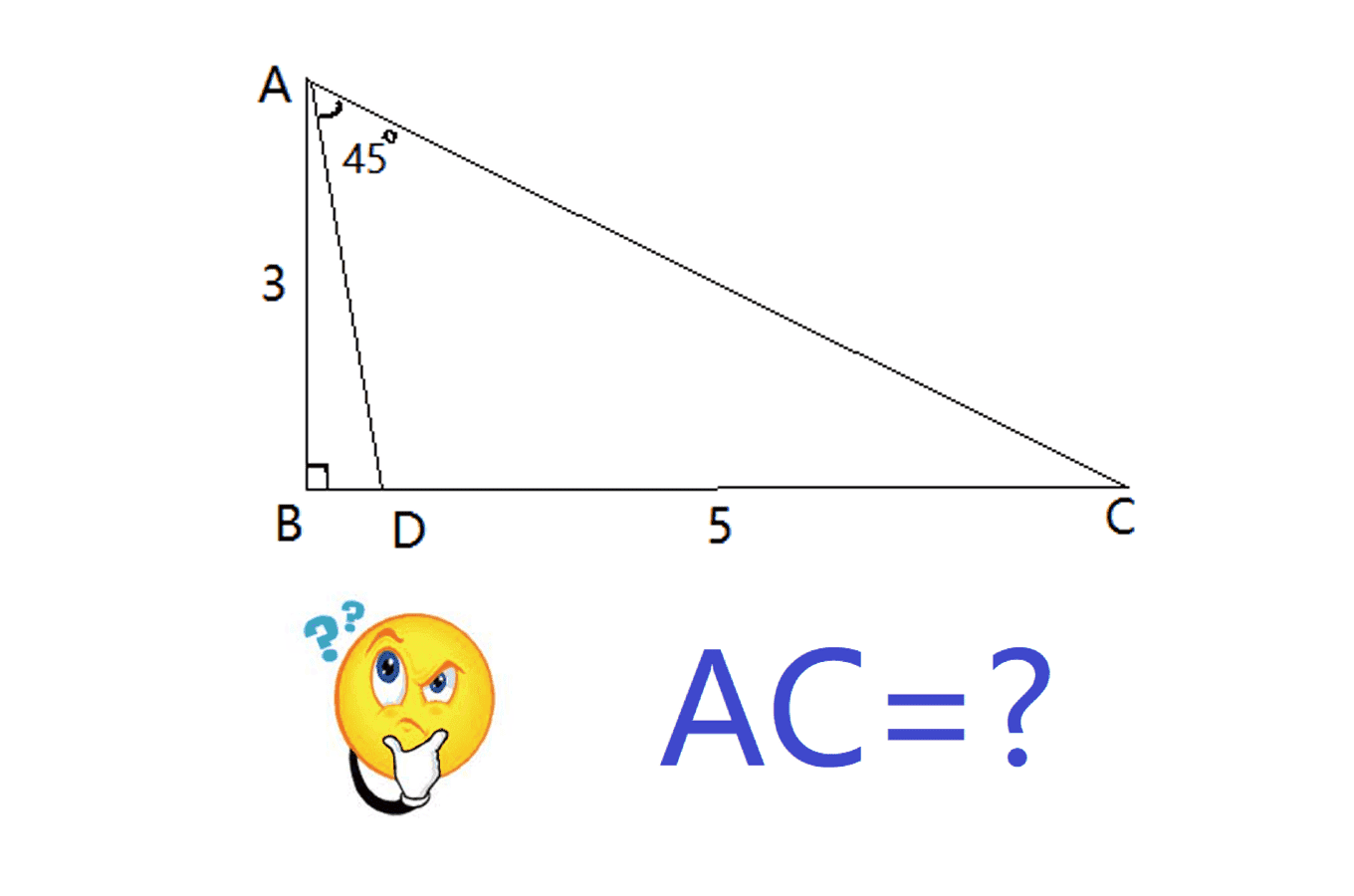# How to Find the Length of the Hypotenuse of a Right Triangle?

• MHB
DrLiangMath
AB=3, DC=5, CAD=$45^o$, AB ⊥ BC. Find the length of AC.Gold Member
MHB
AB=3, DC=5, CAD=$45^o$, AB ⊥ BC. Find the length of AC.

View attachment 11867
It's inefficient but start by defining angle BCA to be C. Then angle CDA = 135 - C. Call x = AC. Then by Law of Sines
$$\displaystyle \dfrac{sin(90)}{x} = \dfrac{sin(45)}{5}$$

$$\displaystyle \dfrac{sin(135 - C)}{x} = \dfrac{sin(45)}{5}$$

This gives a biquadratic in x. But, as I said, it's inefficient. There is probably a better approach.

-Dan

DrLiangMath
Thank you for your response. But we still need one more equation since we have 2 variables x and C.

Gold Member
MHB
Thank you for your response. But we still need one more equation since we have 2 variables x and C.
I gave two equations. The first is the Law of Sines in triangle ABC and the second is the Law of Sines in triangle ADC. Perhaps I should have stated that.

-Dan

Homework Helper
MHB
Hey MathTutoringByDrLiang,

Are you actually looking for an answer to this problem? Or do you have an elegant answer to share?
If the latter, then we might move this thread to the https://mathhelpboards.com/forums/challenge-questions-and-puzzles.28/ subforum.

Btw, at this time I could provide an answer, but it's rather long winded and I'm still looking for a more elegant solution.

Homework Helper
MHB
Here's the best I could find.
\begin{tikzpicture}
%preamble \usetikzlibrary {angles,quotes}
\coordinate[label=left:A] (A) at (0,3);
\coordinate[label=left:B] (B) at (0,0);
\coordinate[label=right:C] (C) at (6,0);
\coordinate[label=below: D] (D) at (1,0);
\draw (B) rectangle +(0.2,0.2);
\draw[thick] (A) -- (B) -- (C) -- cycle (A) -- (D);
\path (A) -- node[ left ] {3} (B) -- node[below] {$y$} (D) -- node[below] {5} (C) -- node[above right] {$x$} (A);
\pic ["$45^\circ$", draw, angle radius=0.4cm, angle eccentricity=2.2,pic text options={shift={(0.1,0)}}] {angle = D--A--C};
\pic ["$135^\circ-C$", draw, angle radius=0.3cm, angle eccentricity=2.2,pic text options={shift={(0.5,0)}}] {angle = C--D--A};
\end{tikzpicture}
Let $x=AC$, which is what we want to find.
Let $y=BD$.

From the law of sines we have:
$$\frac 5{\sin 45}=\frac x{\sin(135-C)} \implies x=5\cdot\frac{\sin(135-C)}{\sin 45}=5\cdot\frac{\sin 135\cos C -\cos 135\sin C}{\sin 45}=5\cdot\frac{\sin 45\cos C +\cos 45\sin C}{\sin 45}=5(\cos C+\sin C) \tag 1$$
From the definitions of cosine and sine:
$$\cos C=\frac{BD+5}{x}=\frac{y+5}{x} \tag 2$$
$$\sin C=\frac 3{x} \tag 3$$
From Pythagoras:
$$x^2 = (y + 5)^2 + 3^2 \tag 4$$

Substitute (2) and (3) into (1) to find:
$$x=5\left(\frac{y+5}x+\frac 3x\right) \implies x^2=5(y+8)\tag 5$$
Substitute in (4):
$$5(y+8) = (y + 5)^2 + 3^2 \implies y^2+5y-6= (y-1)(y+6) = 0 \implies y =1 \tag 6$$
Substitute back into (5):
$$x^2=5(1+8)=45 \implies x=3\sqrt 5$$

Last edited:
DrLiangMath
Hey MathTutoringByDrLiang,

Are you actually looking for an answer to this problem? Or do you have an elegant answer to share?
If the latter, then we might move this thread to the https://mathhelpboards.com/forums/challenge-questions-and-puzzles.28/ subforum.

Btw, at this time I could provide an answer, but it's rather long winded and I'm still looking for a more elegant solution.

I do have a solution to share. Different form the solution given by Klaas van Aarsen, my solution is based on creating new triangles. If you don't mind, I would like post it here for your reference:

Homework Helper
MHB
Just for fun, I've created a TikZ diagram that corresponds to your video.
TikZ is natively supported on this site similar to formulas. Click on the picture to see the $\LaTeX$ code.
\begin{tikzpicture}[scale=2]
%preamble \usepackage{tkz-euclide}
\tkzDefPoint(0,3){A}
\tkzDefPoint(0,0){B}
\tkzDefPoint(6,0){C}
\tkzDefPoint(1,0){D}

\tkzDrawSegments[ultra thick](A,B B,C C,A A,D)

\tkzDefPointWith[orthogonal,K=-1](D,A)
\tkzGetPoint{E}
\tkzDefPointBy[projection=onto B--C](E)
\tkzGetPoint{F}
\tkzDrawSegments[help lines](D,E E,F)

\tkzLabelPoints[ left ](A,B)
\tkzLabelPoints[ right ](C)
\tkzLabelPoints[ below ](D)
\tkzLabelPoints[ below,help lines ](F)
\tkzLabelPoints[ above right,help lines ](E)
\tkzLabelSegment(B,A){3}
\tkzLabelSegment(C,D){5}
\tkzLabelSegment(D,B){$x$}
\tkzLabelSegment[help lines](E,F){$x$}
\tkzLabelSegment[help lines](D,F){3}
\tkzLabelSegment[help lines](F,C){2}

\tkzLabelAngles[pos=1](D,A,C){$45^\circ$}
\tkzMarkRightAngles(A,B,C)
\tkzMarkAngles[size=0.7,mark=none](D,A,C)

\tkzLabelAngles[pos=1,help lines](A,E,D){$45^\circ$}
\tkzMarkAngles[size=0.7,mark=none,help lines](A,E,D)
\tkzMarkAngles[size=0.6,mark=||,help lines](B,A,D F,D,E)
\tkzMarkRightAngles[help lines](A,D,E E,F,D)
\tkzMarkSegments[mark=|,help lines](A,D D,E)
\end{tikzpicture}

DrLiangMath
Wow, it looks so nice! Thank you!